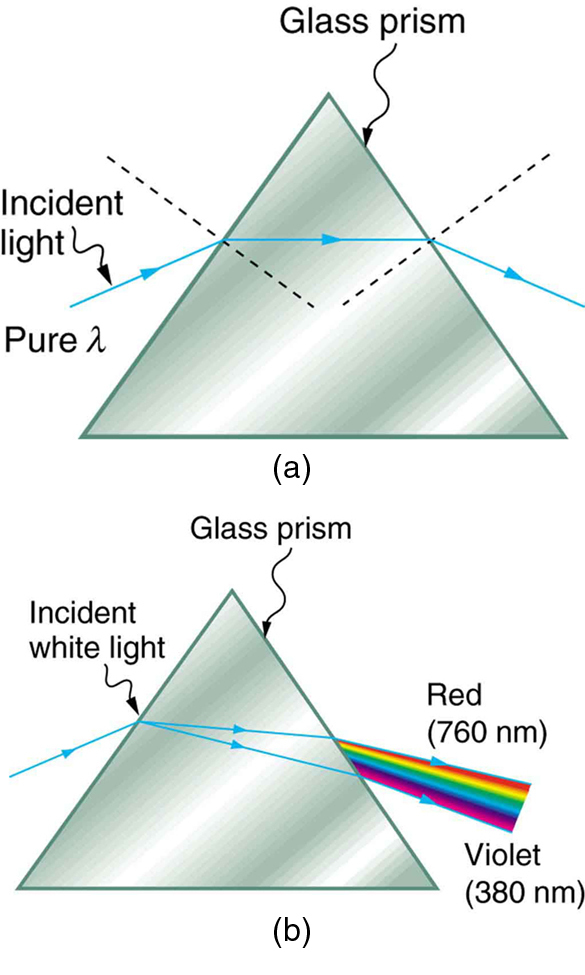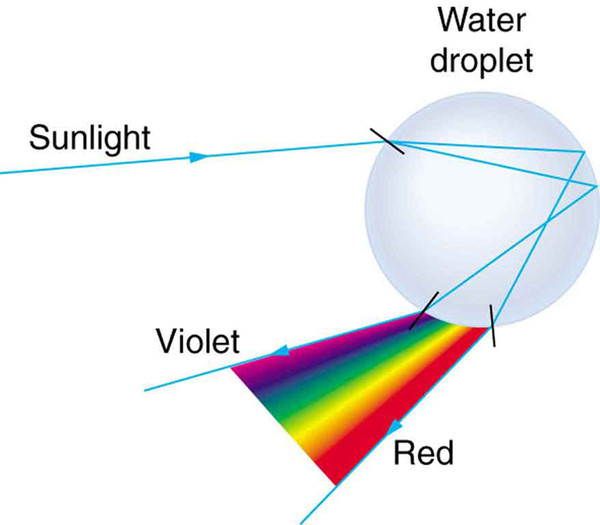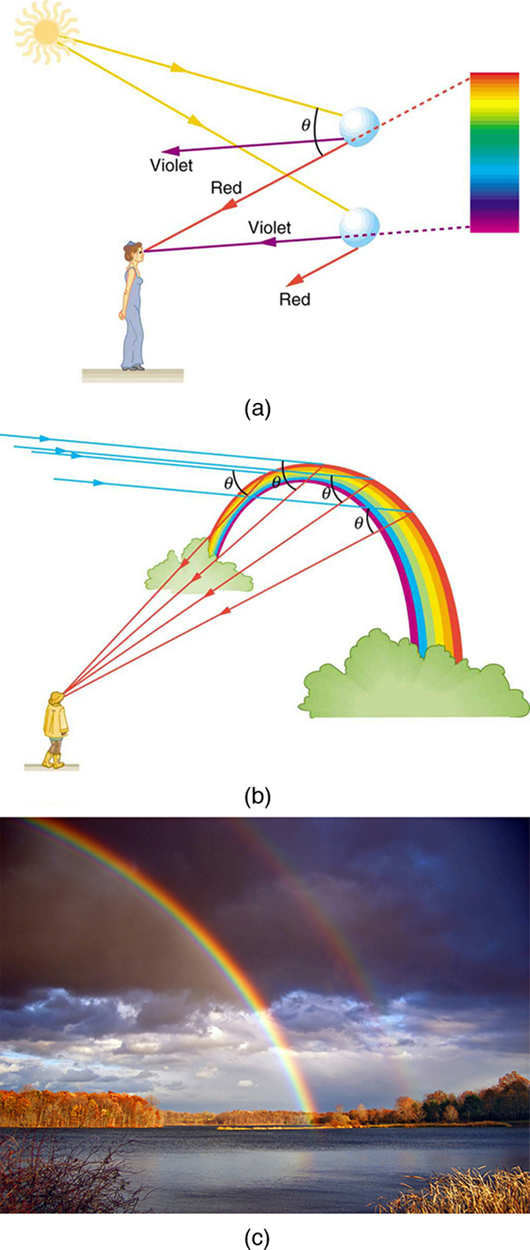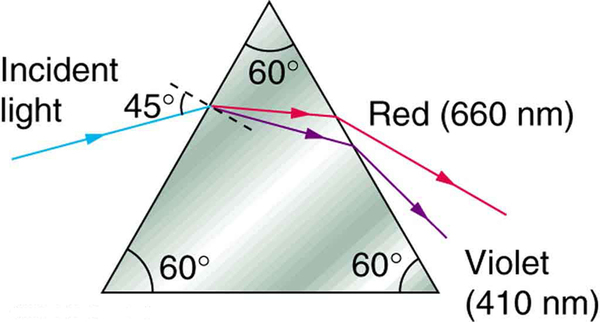# 25.5 Dispersion: the rainbow and prisms  (Page 2/4)

 Page 2 / 4
Index of refraction n In selected media at various wavelengths
Medium Red (660 nm) Orange (610 nm) Yellow (580 nm) Green (550 nm) Blue (470 nm) Violet (410 nm)
Water 1.331 1.332 1.333 1.335 1.338 1.342
Diamond 2.410 2.415 2.417 2.426 2.444 2.458
Glass, crown 1.512 1.514 1.518 1.519 1.524 1.530
Glass, flint 1.662 1.665 1.667 1.674 1.684 1.698
Polystyrene 1.488 1.490 1.492 1.493 1.499 1.506
Quartz, fused 1.455 1.456 1.458 1.459 1.462 1.468(a) A pure wavelength of light falls onto a prism and is refracted at both surfaces. (b) White light is dispersed by the prism (shown exaggerated). Since the index of refraction varies with wavelength, the angles of refraction vary with wavelength. A sequence of red to violet is produced, because the index of refraction increases steadily with decreasing wavelength.

Rainbows are produced by a combination of refraction and reflection. You may have noticed that you see a rainbow only when you look away from the sun. Light enters a drop of water and is reflected from the back of the drop, as shown in [link] . The light is refracted both as it enters and as it leaves the drop. Since the index of refraction of water varies with wavelength, the light is dispersed, and a rainbow is observed, as shown in [link] (a). (There is no dispersion caused by reflection at the back surface, since the law of reflection does not depend on wavelength.) The actual rainbow of colors seen by an observer depends on the myriad of rays being refracted and reflected toward the observer’s eyes from numerous drops of water. The effect is most spectacular when the background is dark, as in stormy weather, but can also be observed in waterfalls and lawn sprinklers. The arc of a rainbow comes from the need to be looking at a specific angle relative to the direction of the sun, as illustrated in [link] (b). (If there are two reflections of light within the water drop, another “secondary” rainbow is produced. This rare event produces an arc that lies above the primary rainbow arc—see [link] (c).)

## Rainbows

Rainbows are produced by a combination of refraction and reflection.Part of the light falling on this water drop enters and is reflected from the back of the drop. This light is refracted and dispersed both as it enters and as it leaves the drop.(a) Different colors emerge in different directions, and so you must look at different locations to see the various colors of a rainbow. (b) The arc of a rainbow results from the fact that a line between the observer and any point on the arc must make the correct angle with the parallel rays of sunlight to receive the refracted rays. (c) Double rainbow. (credit: Nicholas, Wikimedia Commons)

Dispersion may produce beautiful rainbows, but it can cause problems in optical systems. White light used to transmit messages in a fiber is dispersed, spreading out in time and eventually overlapping with other messages. Since a laser produces a nearly pure wavelength, its light experiences little dispersion, an advantage over white light for transmission of information. In contrast, dispersion of electromagnetic waves coming to us from outer space can be used to determine the amount of matter they pass through. As with many phenomena, dispersion can be useful or a nuisance, depending on the situation and our human goals.

## Phet explorations: geometric optics

How does a lens form an image? See how light rays are refracted by a lens. Watch how the image changes when you adjust the focal length of the lens, move the object, move the lens, or move the screen.

## Section summary

• The spreading of white light into its full spectrum of wavelengths is called dispersion.
• Rainbows are produced by a combination of refraction and reflection and involve the dispersion of sunlight into a continuous distribution of colors.
• Dispersion produces beautiful rainbows but also causes problems in certain optical systems.

## Problems&Exercises

(a) What is the ratio of the speed of red light to violet light in diamond, based on [link] ? (b) What is this ratio in polystyrene? (c) Which is more dispersive?

A beam of white light goes from air into water at an incident angle of $\text{75}\text{.}0º$ . At what angles are the red (660 nm) and violet (410 nm) parts of the light refracted?

$\text{46}\text{.}5º\text{, red; 46}\text{.}0º\text{, violet}$

By how much do the critical angles for red (660 nm) and violet (410 nm) light differ in a diamond surrounded by air?

(a) A narrow beam of light containing yellow (580 nm) and green (550 nm) wavelengths goes from polystyrene to air, striking the surface at a $\text{30}\text{.}0º$ incident angle. What is the angle between the colors when they emerge? (b) How far would they have to travel to be separated by 1.00 mm?

(a) $0\text{.}\text{043º}$

(b) $1\text{.}\text{33 m}$

A parallel beam of light containing orange (610 nm) and violet (410 nm) wavelengths goes from fused quartz to water, striking the surface between them at a $\text{60}\text{.}0º$ incident angle. What is the angle between the two colors in water?

A ray of 610 nm light goes from air into fused quartz at an incident angle of $\text{55}\text{.}0º$ . At what incident angle must 470 nm light enter flint glass to have the same angle of refraction?

$\text{71.3º}$

A narrow beam of light containing red (660 nm) and blue (470 nm) wavelengths travels from air through a 1.00 cm thick flat piece of crown glass and back to air again. The beam strikes at a $\text{30}\text{.}0º$ incident angle. (a) At what angles do the two colors emerge? (b) By what distance are the red and blue separated when they emerge?

A narrow beam of white light enters a prism made of crown glass at a $\text{45}\text{.}0º$ incident angle, as shown in [link] . At what angles, ${\theta }_{\text{R}}$ and ${\theta }_{\text{V}}$ , do the red (660 nm) and violet (410 nm) components of the light emerge from the prism?This prism will disperse the white light into a rainbow of colors. The incident angle is 45 . 0º , and the angles at which the red and violet light emerge are θ R and θ V size 12{q rSub { size 8{V} } } {} .

$\text{53.5º}\text{, red;}$ $\text{55.2º}\text{, violet}$

full meaning of GPS system
how to prove that Newton's law of universal gravitation F = GmM ______ R²
sir dose it apply to the human system
prove that the centrimental force Fc= M1V² _________ r
prove that centripetal force Fc = MV² ______ r
Kaka
how lesers can transmit information
griffts bridge derivative
below me
please explain; when a glass rod is rubbed with silk, it becomes positive and the silk becomes negative- yet both attracts dust. does dust have third types of charge that is attracted to both positive and negative
what is a conductor
Timothy
hello
Timothy
below me
why below you
Timothy
no....I said below me ...... nothing below .....ok?
dust particles contains both positive and negative charge particles
Mbutene
corona charge can verify
Stephen
when pressure increases the temperature remain what?
remains the temperature
betuel
what is frequency
define precision briefly
CT scanners do not detect details smaller than about 0.5 mm. Is this limitation due to the wavelength of x rays? Explain.
hope this helps
what's critical angle
The Critical Angle Derivation So the critical angle is defined as the angle of incidence that provides an angle of refraction of 90-degrees. Make particular note that the critical angle is an angle of incidence value. For the water-air boundary, the critical angle is 48.6-degrees.
okay whatever
Chidalu
pls who can give the definition of relative density?
Temiloluwa
the ratio of the density of a substance to the density of a standard, usually water for a liquid or solid, and air for a gas.
Chidalu
What is momentum
mass ×velocity
Chidalu
it is the product of mass ×velocity of an object
Chidalu
how do I highlight a sentence]p? I select the sentence but get options like copy or web search but no highlight. tks. src
then you can edit your work anyway you want
Wat is the relationship between Instataneous velocity
Instantaneous velocity is defined as the rate of change of position for a time interval which is almost equal to zero
AstronomyByBy Jazzycazz JacksonBy Richley CrapoBy RhodesBy Stephen VoronBy Angelica LitoBy Richley CrapoBy Mistry BhaveshBy Brooke DelaneyBy Sean WiffleBoyBy Hope Percle